# Square CTF 2018 - Gates of hells WriteUp

This is a reverse challenge.
As we will see, this is probably a code written directly in assembly, and not some C code compilated.
Since the code is quite short, we will be able to read it all.

First, we try running the binary: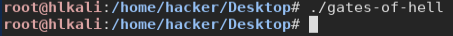Nothing happens.

Have a look inside: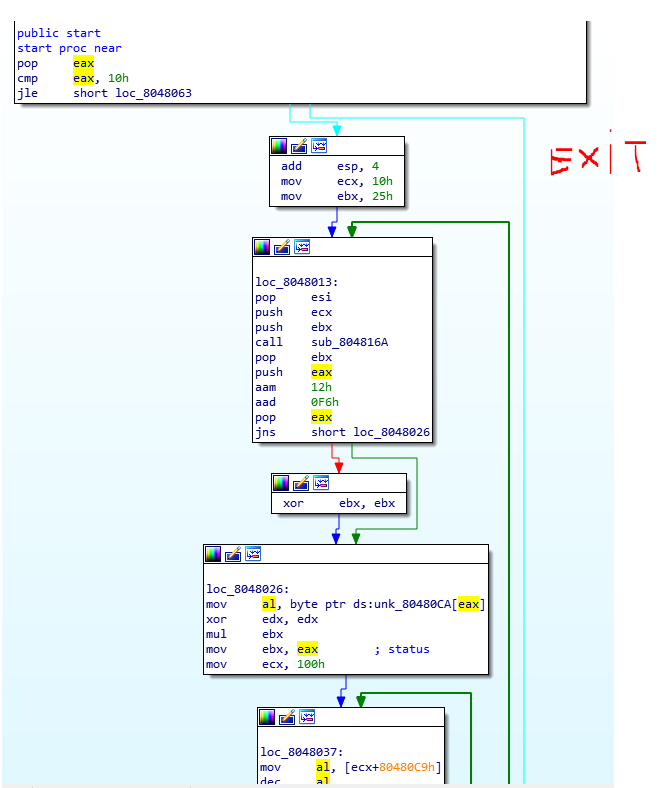We can see that during the first 3 instruction
a pop takes the number of arguments and compares it with 0x10 (16).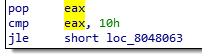So we need to provide 15 arguments? (the first is the name of the program).

Ok, 15 arguments, but when will we win?
we can find this function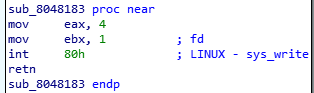which is a system call to a print. (which is probably our win function).

as we can see this function will be called if ebx will be equal at 0x29A (666)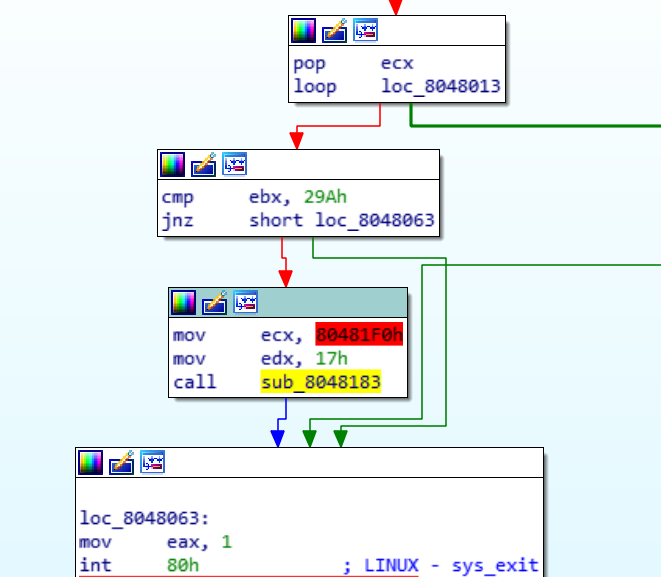Otherwise, it will just exit

Ok, we need to understand how to make ebx become equal to 0x29A.

Now we follow the code flow after the argument check: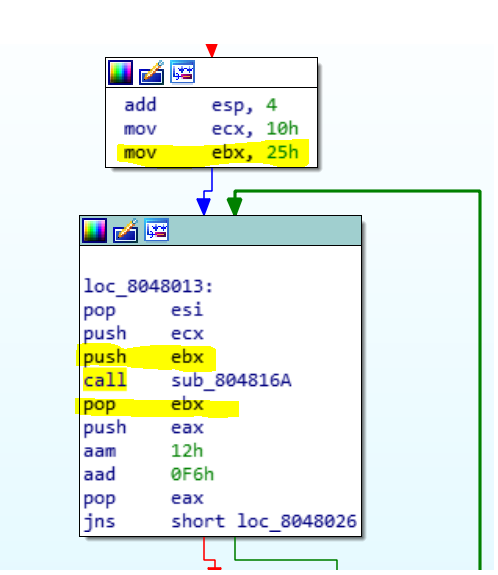A function is called and the value of ebx which start with 0x25 is stored into the stack and retrieved after the function.
We should investigate this function.

This is the function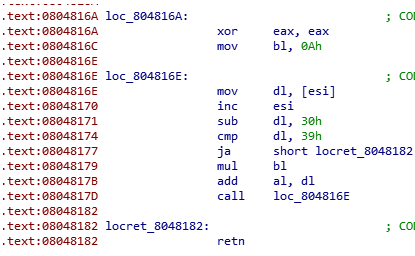Running with gdb we can see that [esi] will contain our input (the 15 arguments provided), and we also notice that every argument is treated as 2 bytes. This means that if our argument is ‘A’ this function will take 0x41 and 0x0 at the second iteration.
The 3rd iteration will always not respect the jump condition, so will exit.

So, we can reassume that instruction as:

This mean that if we alwaise provide chars >=0x70 we will have

good, that’s function is quite predictable then, but what is this for?

Here is the “main” functionlooking at the entirety of the code we can understand better what it does.

1) The previous function is called, its value will be stored in EAX
2) The result of the function is “stored” in the stack and some strange operations are performed, we will look at that later, after this the value of EAX is retrieved.
3) If those “strange operation” menage to set the sign flag, ebx will be set to 0
4) eax (the result of the function) is used as index of a vector, the content of v[eax] is moved into al (lower part of eax of 1 byte)
5) A mul is performed, so it will exec ebx * vet[eax]
6) the result is stored into ebx again.
7) Here ecx is set at 100. at the instruction n. 10 we can see a loop, so the instruction between point 7 and 10 repeats 100 times.
8) vet2[i] (where ‘i’ is ecx) is moved into al, and his value will be decreased.
9) if the result will be >= 0 al will be stored back into vet2[i].
10) end of the first loop
11) this an external loop, which loops for 15 times, one for every argument.

So, we want ebx to become equal to 0x29A.
it starts with 0x25.
For every cycle ebx = ebx * vet[eax] (where eax is the return value of our first function).
And, after that, all the value of vet will decrease by 1
Ok, we are close.

Now we want to:

1) Create some code that giving the index needed return to us the argument to provide as input (reverse the first function)

2) have a better look at the instruction at point 3) which could make ebx to reset (and we will lose)

3) Choose the index of the vector, so that their product will be equal to 18 (since ebx start to 37 (0x25) 37*18 = 666 (0x29A))

The first task is quite easy.

Since

we can write a simple code that could find the correct value of ch1 and ch2 giving a result.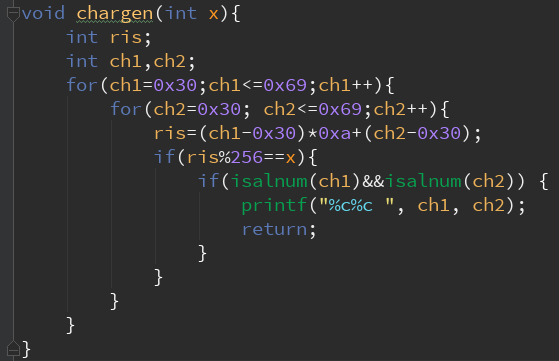Now we want to avoid that ebx will be reset.
In order to do that we want eax be such that

won’t provide a negative resoult.

We can find the behavior of those 2 instrctions

The first will transform al into a bcd value in base 0x12
the second will do the reverse, but with a different base (0xF6)

Ok, luckily this site also provides a pseudocode, so we could easily write a C function that simulates it.

Here it is: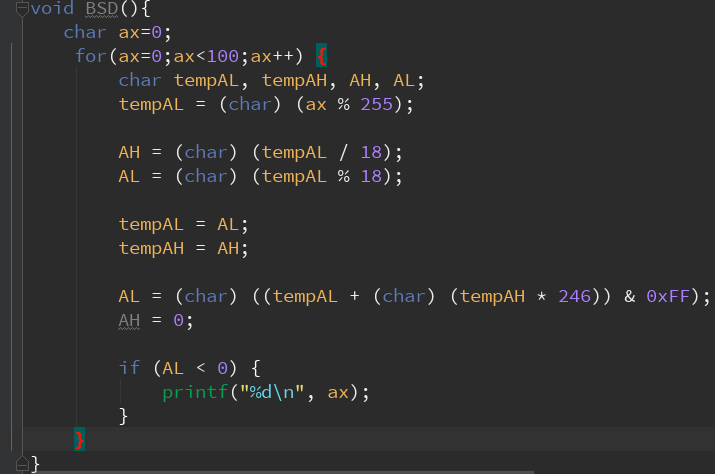This will provide all the “bad values” (which are a lot)

Great.

Using gdb we can look at the initial stat of the vecotor:

If we esclude the bad index from provided by the script of the task 2 we get:

Now we could wrote a script that chose the right value to make ebx equal to 0x29A
But in our case @matpro98 found by hand one solution:

The index where:
34,15,30,17,4,16,28,14,33,5,5,12,9,7,3,6

Now we just want to give those index to the function that transforms them into the input and we find:
0R 15 0N 0A 04 16 0L 14 0Q 05 05 12 09 07 03 06

which is the correct input to make the program print the flag!